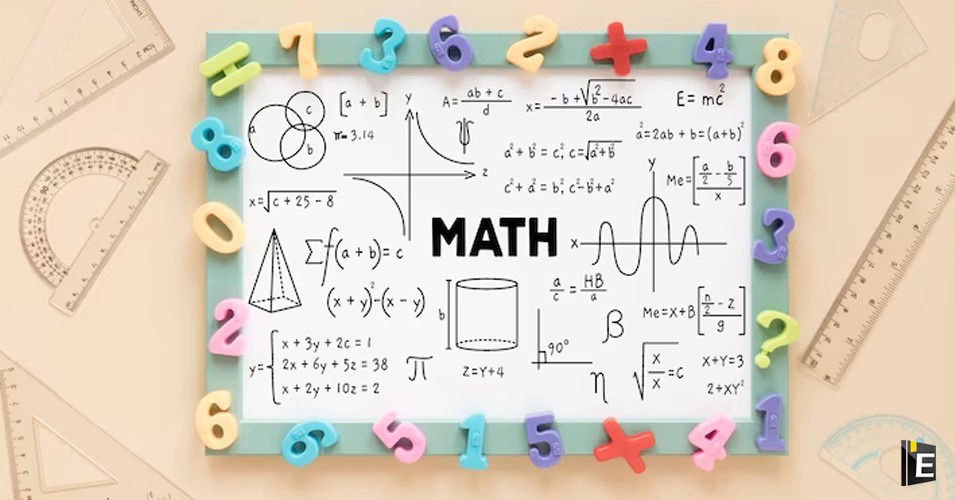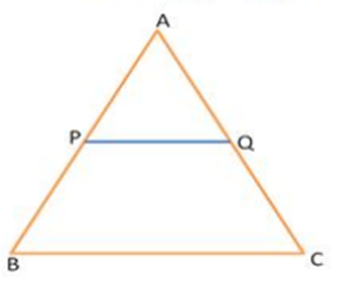• Save

# Volume and Surface Area Questions Quiz with Answers

NEWAre you ready to master your geometry skills? Take our Volume and Surface Area Questions Quiz and put your knowledge to the test! This Volume and Surface Area Questions Quiz with Answers blog features a range of challenging math problems, all centred around calculating volumes and surface areas of 3D shapes.

## Volume and Surface Area Questions

Whether you're a student looking to improve your math skills or someone preparing for a job interview that involves geometry, this Volume and Surface Area Questions Quiz with Answers blog is perfect for you. Our carefully curated questions cover various geometric shapes, including cubes, cylinders, spheres, and more.

Also, Read Latest Current Affairs Questions 2023: Current Affairs Today

"Put your knowledge to the test and excel with our General Knowledge Mock Test and Current Affairs Mock Test!"

## Volume and Surface Area Questions Quiz with Answers

Q :

If a chord of length 30 cm is at a distance of 8 cm from the center of the circle, then the length of the chord of the same circle which is at a distance of 15 cm from the center is equal to

(A) 12 cm

(B) 18 cm.

(C) 16 cm

(D) 20 cm.

Q :

The diagonals of a rhombus are 24 cm. and 10 cm. The perimeter (in cm) of this rhombus is-

(A) 54

(B) 68

(C) 52

(D) 54

Q :

The length, breadth and height of a room is 21 metres, 12 metres and 16 metres. What will be the length of the largest rod that can be placed in that room?

(A) 32 metres

(B) 25 metres

(C) 31 metres

(D) 29 metres

Q :

Area of a circle inscribed in a square is 308 cm2.The length of the diagonal of the square is

(A) 16 cm

(B) 28 cm

(C) 24 cm

(D) 22 cm

Q :

The ratio of the areas of two isosceles triangles having the same vertical angle is 1 : 9.The ratio of their heights is -

(A) 1 : 3

(B) 1 : 9

(C) 3 : 1

(D) 1 : 81

Q :

The curved surface area of cylindrical pillar is $${264 m^{2}}$$ and its volume is $${924 m^{3}}$$. Find the ratio of its diameter to its height.

(A) 3 : 7

(B) 7 : 3

(C) 6 : 7

(D) 7 : 6

Q :

If the height and the radius of the base of a cone are both doubled, the volume of the cone becomes _______ of its previous volume.

(A) 4 times

(B) 8 times

(C) 3 times

(D) 6 times

Q :

In a triangle, ABC, P and Q are points on AB and AC, respectively, such that AP = 1 cm, PB = 3 cm, AQ = 1.5 cm, and CQ = 45 cm. If the area of ΔAPQ is 12 $${{cm^2}}$$, then find the area of BPQC.(A) $${{192cm^2}}$$

(B) $${{182cm^2}}$$

(C) $${{190cm^2}}$$

(D) $${{180cm^2}}$$

Q :

The diameter of a circle is equal to the perimeter of a square whose area is 3136 sq cm. What is the circumference of the circle?

(A) 352 cm

(B) 704 cm

(C) 394 cm

(D) None of these

Q :

If the length of the diagonal of a square is $${ 10\sqrt{2}}$$ cm, then what will be the area of the square?

(A) 200 cm2

(B) 100 cm2

(C) $${ 100\sqrt{2}}$$

(D) 50 cm2